## Saturday, 9 August 2014

### CHAPTER 1 - 3-D Geometry

#### INTRODUCTION : BASIC FORMULA

we need three non-co planar directions as our reference axis to specify the position of any point. It is most convenient to take the three non-coplanar directions (denoted conventionally by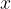$x$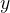$y$ and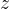$z$ directions) as being mutually perpendicular to each other.Such a reference axes is termed a rectangular coordinate axis. Any point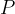$P$ in space can now be specified with respect to this frame by specifying the components (coordinates) of$P$ along each axis.
For example, in the figure below,the coordinates of$P$ along the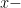$x -$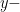$y -$ and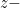$z -$axis are given to be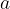$a$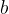$b$ and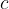$c$. Thus,$P$ can be specified as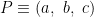$P \equiv \left( {a,\,\,b,\,\,c} \right)$
In two dimensions, two coordinates were sufficient to uniquely determine any point; in three dimensions, we need three coordinates.
Note that a two dimensional coordinate axes divides the plane into four quadrants; a three dimensional coordinate axes will divide the space into eight “compartments” known as octants. As an elementary exercise, write down the coordinates of some arbitrary points in each of the eight octants.

#### DISTANCE FORMULA

Let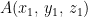$A({x_1},\,{y_1},\,{z_1})$ and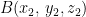$B({x_2},\,{y_2},{z_2})$ be two arbitrary points. We need to find the distance between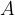$A$ and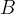$B$ in terms of the coordinates of$A$ and$B$. Observe the following diagram carefully: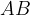$AB$ is simply the length of the diagonal of the cuboid drawn (as depicted above):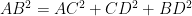$A{B^2} = A{C^2} + C{D^2} + B{D^2}$$A{B^2} = {\left( {{x_1} - {x_2}} \right)^2} + {\left( {{y_1} - {y_2}} \right)^2} + {\left( {{z_1} - {z_2}} \right)^2}$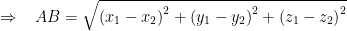$\Rightarrow\,\,\,\,\,{AB = \sqrt {{{\left( {{x_1} - {x_2}} \right)}^2} + {{\left( {{y_1} - {y_2}} \right)}^2} + {{\left( {{z_1} - {z_2}} \right)}^2}} }$
This is the distance formula for three dimensions. It has a form exactly similar to the distance formula for two dimensions.
As an example, let$A \equiv \left( {1,\,\,2,\,\,3} \right),\,\,B \equiv \left( {3,\,\,2,\,\,1} \right)$ and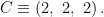$C \equiv \left( {2,\,\,2,\,\,2} \right).$ We have,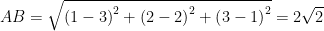$AB = \sqrt {{{\left( {1 - 3} \right)}^2} + {{\left( {2 - 2} \right)}^2} + {{\left( {3 - 1} \right)}^2}} = 2\sqrt 2$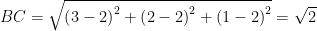$BC = \sqrt {{{\left( {3 - 2} \right)}^2} + {{\left( {2 - 2} \right)}^2} + {{\left( {1 - 2} \right)}^2}} = \sqrt 2$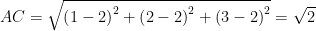$AC = \sqrt {{{\left( {1 - 2} \right)}^2} + {{\left( {2 - 2} \right)}^2} + {{\left( {3 - 2} \right)}^2}} = \sqrt 2$
Incidentally,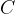$C$ is the mid point of$AB$ since$AC + BC = AB$ and$AC = BC$.
 Example: 1
 Find the locus of the point$P$ equidistant from the four points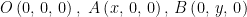$O\left( {0,\,0,\,0} \right),\,\,A\left( {x,\,0,\,0} \right),\,B\left( {0,\,y,\,0} \right)$ and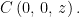$C\left( {0,\,0,\,z} \right).$
 Solution: 1
Let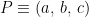$P \equiv \left( {a,\,b,\,c} \right)$ We have,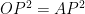$O{P^2} = A{P^2}$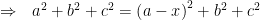$\Rightarrow \,\,\,\, {a^2} + {b^2} + {c^2} = {\left( {a - x} \right)^2} + {b^2} + {c^2}$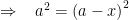$\Rightarrow \,\,\,\,\, {a^2} = {\left( {a - x} \right)^2}$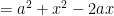$= {a^2} + {x^2} - 2ax$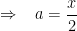$\Rightarrow \,\,\,\,\, a = \dfrac{x}{2}$
Similarly,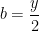$b = \dfrac{y}{2}$ and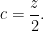$c = \dfrac{z}{2}.$ Thus, the point P is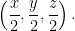$\left( {\dfrac{x}{2},\dfrac{y}{2},\dfrac{z}{2}} \right).$
 Example: 2
 Find the locus of a point$P$ which moves so that its distances from the points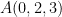$A(0,2,3)$ and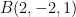$B(2, - 2,1)$ are always equal.
 Solution: 2$P$ will obviously lie on the perpendicular bisector of$AB$. Let the co-ordinates of$P$ be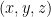$(x,y,z)$.
Therefore,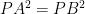$P{A^2} = P{B^2}$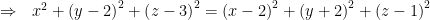$\Rightarrow \,\,\,\, {x^2} + {\left( {y - 2} \right)^2} + {\left( {z - 3} \right)^2} = {\left( {x - 2} \right)^2} + {\left( {y + 2} \right)^2} + {\left( {z - 1} \right)^2}$$\Rightarrow \,\,\,\, x - 2y - z + 1 = 0$
This is the required locus of$P$ (if you think carefully, it is a plane)